Deutsche VersionThe Formula WheelFormulas of Acoustics (Audio)
for sound pressure p, particle velocity v, specific acoustic impedance of air Z, sound intensity I or J
Magic circle − Formulas for calculating any combination of acoustical units – audio engineering formulas

 Principles of Acoustic Law and Sound Equations in Analogy (Relationship) to the Ohm's Law. Formulary and Audio Equations Formula Wheel ♥ Important Formulas of Acoustics + Audio of Sound + Noise Acoustical formulas as circle diagram (pie chart)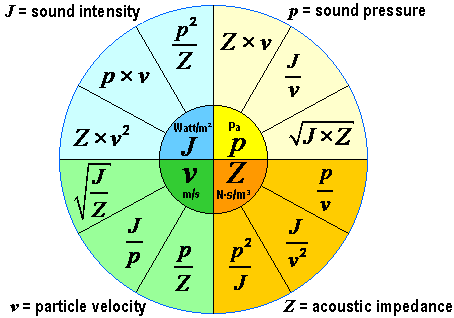In acoustics and audio engineering sound pressure, air particle velocity, and acoustic impedance are sound field quantities. The sound intensity is a sound energy quantities.

 Sound pressure p = Z × v = J / v = √(J × Z) in Pa             Particle velocity v = p / Z = J / p = √(J / Z) in m/s Acoustic impedance Z = p / v = J / v2 = p2 / J in N·s/m3      Sound intensity J = p × v = Z × v2 = p2 / Z in W/m2

 Specific acoustic impedance Z0 = ρ × c = p / v in N·s/m3. As characteristic impedance of air we use the round value Z0 = 400 N·s/m³. Then the "sound level" as a decibel value is exactly the same of the sound pressure level and the sound intensity level. Conversion: Sound pressure to Sound intensity and vice versa   Specific acoustic impedance of air at 20°C is Z0 = 413 N·s/m³. Density of air ρ in kg/m3 Speed of sound c = λ × f in m/s Sound power (acoustical power) Pac = J × A = p × v × A in W Area A = 4π × r2 in m2 Sound intensity J = Pac / A =  Pac/ 4π × r2 in W/m2 Particle velocity v = √(E / ρ) in m/s     E  =Sound energy density Particle displacement ξ = p / (ω × ρ0 × c) in m (RMS) Angular frequency ω = 2π × f   Amplitude of sound waves (sound amplitudes) and audio signals

Acoustics, sound, and vibrations − Acoustic equivalent for ohm's law

Electric current, electric power, electricity − Formulas and calculations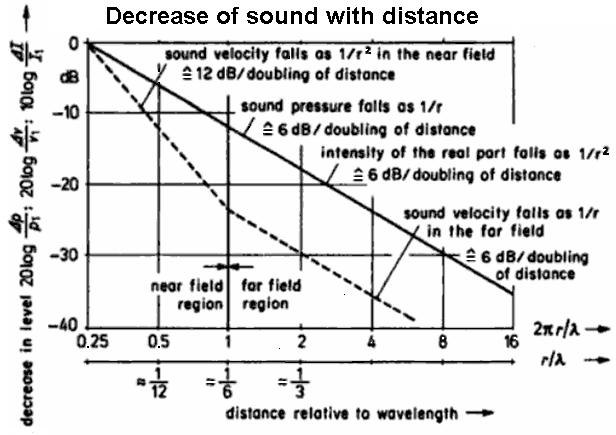Pressure, velocity, and intensity of the sound field near to and distant from a spherical radiator of the zeroth order

Inverse distance law p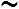1/r for sound pressure                 Inverse square law J1/r² for acoustic intensity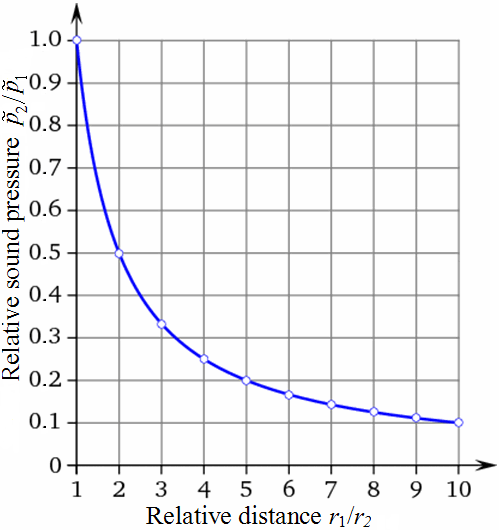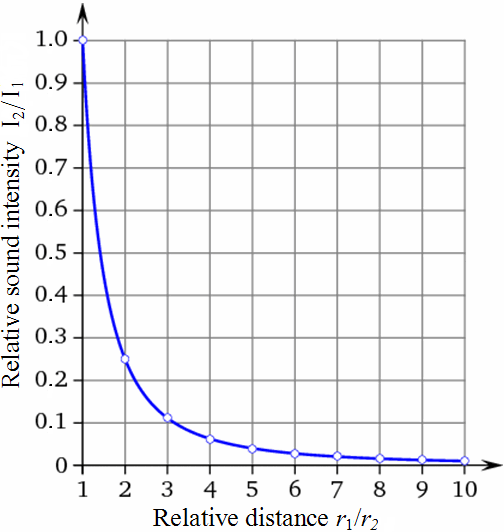Sound pressure, Sound intensity and their Levels

 The following calculator shows the often desired direct conversion of sound pressure to sound intensity and vice versa with the specific acoustic impedance of air Z0 = 400 N·s/m3.

 To use the calculator, simply enter a value. The calculator works in both directions of the ↔ sign.

 Sound field quantity Sound energy quantity Sound pressure p (air) Pa (pascal) ↔ Sound intensity I (air) W/m2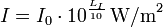Reference sound pressure p0 = 20 μPa = 2 × 10−5 Pa      Reference intensity I0 = 1 pW/m2 = 10−12 W/m2 Specific acoustic impedance of air Z0 = 400 N·s/m3   Sound pressure p = √ (I × Z0)   Intensity I = p2 / Z0
 Sound field quantity Sound energy quantity Sound pressure level Lp (air) dB (decibel) ↔ Sound intensity level LI (air) dB (decibel)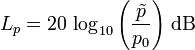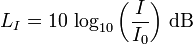Reference sound pressure p0 = 20 μPa = 2 × 10−5 Pa      Reference intensity I0 = 1 pW/m2 = 10−12 W/m2 The same "sound level" in dB at Specific acoustic impedance of air Z0 = 400 N·s/m3
 While the distance dependent sound pressure level in the air is matched with the sound intensity level when a reference sound characteristic impedance Z0 =  400 N·s/m³ is chosen, this is not the case with the distance independent sound power level.

Sound pressure and
Sound pressure levelNote: The radiated sound power (sound intensity) is the cause and the sound pressure is the effect, where the sound engineer is particularly interested in the effect. The effect of temperature and sound pressure: Sound pressure and Sound power – Effect and Cause.

 Acousticians and sound protectors ("noise fighters") need the sound intensity (acoustic intensity) – but sound engineers and sound designers ("ear people") don't need that sound energy quantity. The eardrums (tympanic membranes) of our hearing and the diaphragms of the microphones are effectively moved by the sound pressure or the sound pressure level. See also: SPL meter.

 If you are a technician checking the sound quality by listening with your hearing, think of the sound waves that move your eardrums by the effect of the sound pressure as sound field size. That is why there is the advice: In sound recording try to avoid the use of sound power and sound intensity as sound energy sizes.   How many decibels (dB) is the sound energy W = I×t×A in J = W×s? This question is asked quite rare. For calculations we use more the following sound energy sizes: Sound energy density w or E = I / c in J/m3, sound intensity I = Pac / A in W/m2, and sound power Pac in W = J/s and their corresponding levels.

Sound waves move our eardrums (tympanic membranes).
But which sound quantity produces this effect?Sound pressure and Sound power – Effect and Cause   Sound power is the cause - but sound pressure produces the audible effect.

How to measure sound pressure?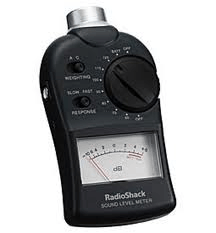The question: "Is noise level really the sound intensity level?" will be differently answered by musicians and sound engineers as "ear people" than by acousticians. We measure the sound of music with a sound level meter (SPL meter) and our eardrums are effectively moved by the sound pressure or its level. A sound engineer thinks that a sound level has mainly to do with the sound pressure deviations. Acousticians as "sound fighters" have a slightly different point of view. They mainly like to calculate the energy (power) of the sound.

 Neither the sound power Pac nor the sound power level LW decreases when the distance is in doubled. Why is this so? The sound power level quantifies the totally radiated sound energy from an object. The noise power level is independent of the distance to the object, the surrounding area and other influences.   Notice: The psychoacoustic subjective sensations of loudness do not belong to those predictable and measurable sound quantities; see:   Correlation between volume level in phone and loudness in sone   We feel and judge sound events as: − exposure duration − spectral composition − temporal structure − sound level − information content − subjective mental attitude

 In sound engineering there is no Power matching or Impedance matching. In audio we use only Voltage bridging or high Impedance bridging.

Sound pressure p = v × Z0      (Acoustic ohm's law)
Sound pressure = particle velocity × acoustic impedance

Please enter two values, the third value will be calculated.
 Sound pressure p PaParticle velocity v m/s Acoustic impedance Z0 N·s/m³ p = v × Z0                    v = p ⁄ Z0                    Z0 = p ⁄ v
Specific acoustic impedance of air is Z = 413 N·s/m³ at 20°C.

Sound intensity J = p × v      (Acoustic power law)
Sound intensity = sound pressure × particle velocity

Please enter two values, the third value will be calculated.
 Sound intensity J W/m²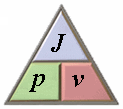Sound pressure p Pa Particle velocity v m/s J = p × v                    p = J ⁄ v                    v = J ⁄ p
Acoustic impedance Z0 = ρ × c
Acoustic impedance of air = density of air × speed of sound in air

Please enter two values, the third value will be calculated.
 Acoustic impedance Z0 N·s/m³Density of air ρ kg/m³ Speed of sound c m/s Z0 = ρ × c                    ρ = Z0 ⁄ c                    c = Z0 ⁄ ρ
Acoustic impedance of air Z0 = 413 N·s/m³ at 20°C.
Density of air ρ = 1.204 kg/m³ at 20°C.

Temperature Dependence of Physical Quantities

Sound pressure is not intensity

 Differentiate: Sound pressure p is a "sound field size" and sound intensity I is a "sound energy size". In teachings these terms are not often separated sharply enough and sometimes are even set equal. But I ~ p2.

Changing of sound power with distance is nonsense

 Question: How does the sound power decrease with distance"? Answer: "April fool - The sound power does not decrease (drop) with distance from the sound source."   Levels of sound pressure and levels of sound intensity decrease equally with the distance from the sound source. Sound power or sound power level has nothing (!) to do with the distance from the sound source. Thinking helps: A 100 watt light bulb has in 1 m and in 10 m distance really always the same 100 watts, which is emitted from the lamp all the time. Watts don't change with distance.   A frequent question: "Does the sound power depend on distance?" The clear answer is: "No, not really."   We consider sound fields in air which are described by the scalar quantity p (sound pressure) and the vector quantity v (sound velocity) as sound field quantities.

Acoustic Units of Measurement

 Sound Quantity Name Abbreviation Basic Units 1) Wavelength λ metre m m 2) Frequency f hertz  kilohertz Hz  kHz 1/s cycles per second   (~) 1 kHz = 1000/s 3) Period T second  millisecond s  ms s 1 ms = 10−3 s 4) Speed of sound c metre/second m/s m/s 5) Sound pressure p pascal  micropascal Pa = N/m2  µPa kg/m·s2 newton/m2  1 µPa = 10−6 Pa 6) Sound intensity I watt/m2 W/m2 kg/s3 joule/s·m2 7) Sound power Pac watt W kg·m2/s3 joule/s

Attributes of Sound

 Physical Perceptual amplitude loudness frequency pitch spectrum timbre duration length